What is a number line? A number line is a straight line which marks numbers at intervals, they are used to help children with various mathematical calculations.

Here is an example:

Question: What is 7 – 5 ?  Children can use the number line by placing their finger on the number 7 and then counting down 5 until they get to the answer, number 2.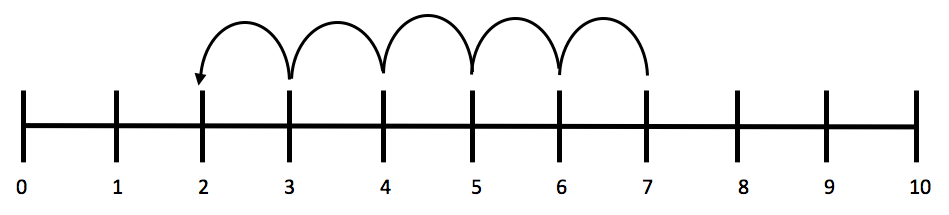Learning to add and subtract using a number line is helpful for children, as it enables them to visualise the calculation by counting up or down the line.

Number lines do not have to start at 0, they can be used to mark out any numbers which are relevant to a question.

## When are children taught to use number lines?

From Reception onwards, children will be familiar with number lines, as teachers will use them to show children the order of numbers and how to count up and down.

Children may also use them In Year 1, when they learn how to count in 2s, 5s and 10s. A good understanding of addition will help children when they start learning their times tables in Year 2.

One way that teachers start introducing times tables is by using number lines to demonstrate multiplications. For example:

To work out the answer to 3 x 3, children can use a number line to count up in 3s three times until they get the answer 9.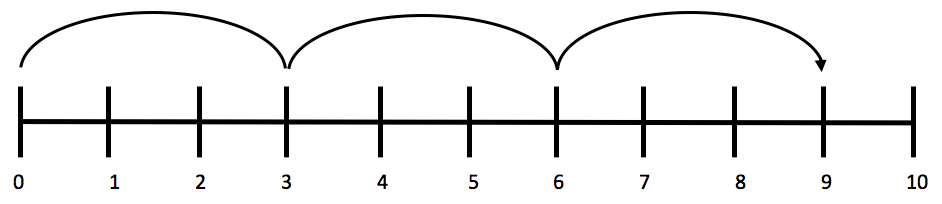In a similar way, number lines can also help children learn how to divide. For example:

To work out 16 ÷ 4, children can place their finger over the number 16 and count backwards in sets of 4, marking the number line each time. Once they have got to 0 they can then count how many marks they made on the line, and they will get the answer 4.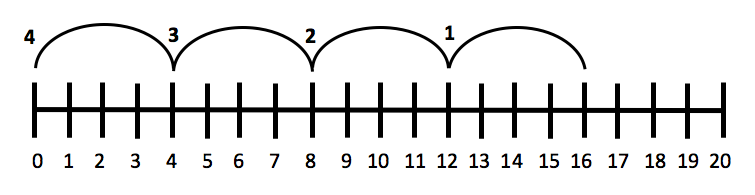For harder subtraction questions, such as 93 – 38, teachers may show children how to use the jump method. The benefit of this method is that it shows children that a subtraction question is just asking you the difference between two numbers.

To work out the calculation above on a number line, the smaller number is put at the start, with the larger number at the end. Then children can jump up in 10s until there is a number less than 10 left to add: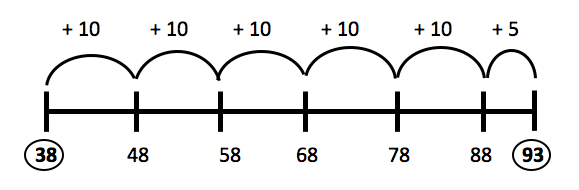After doing this, children simply need to add up all the numbers from each jump they made:

10 + 10 + 10 + 10 + 10 + 5 = 55        (93 - 38 = 55)

During Years 4 to 6, teachers will use number lines to demonstrate negative numbers, decimal numbers and fractions. Here are some examples of how number lines will be used: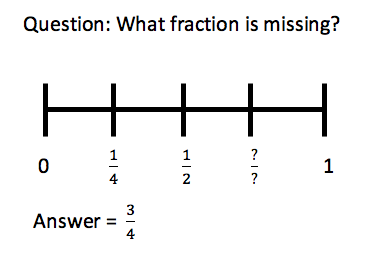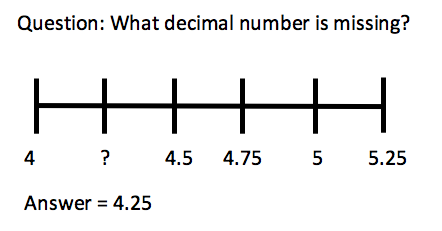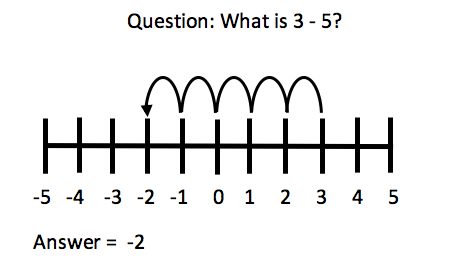## How does Learning Street help children with number lines?

Learning Street will introduce number lines to the children slowly. This will be combined with regular practice in order that it is understood before then beginning to develop their knowledge and extend it further, as well as constant revision so that it isn’t forgotten.

## Our Courses

Click through to review the courses we have available

##### Get Started

Learning Street structured courses make home study easier and more successful. There's no need to wonder which books to buy or what to do next. Everything is planned for you.

• Proven to deliver excellent results
• Used by families, private tutors and schools
• Fully planned and structured